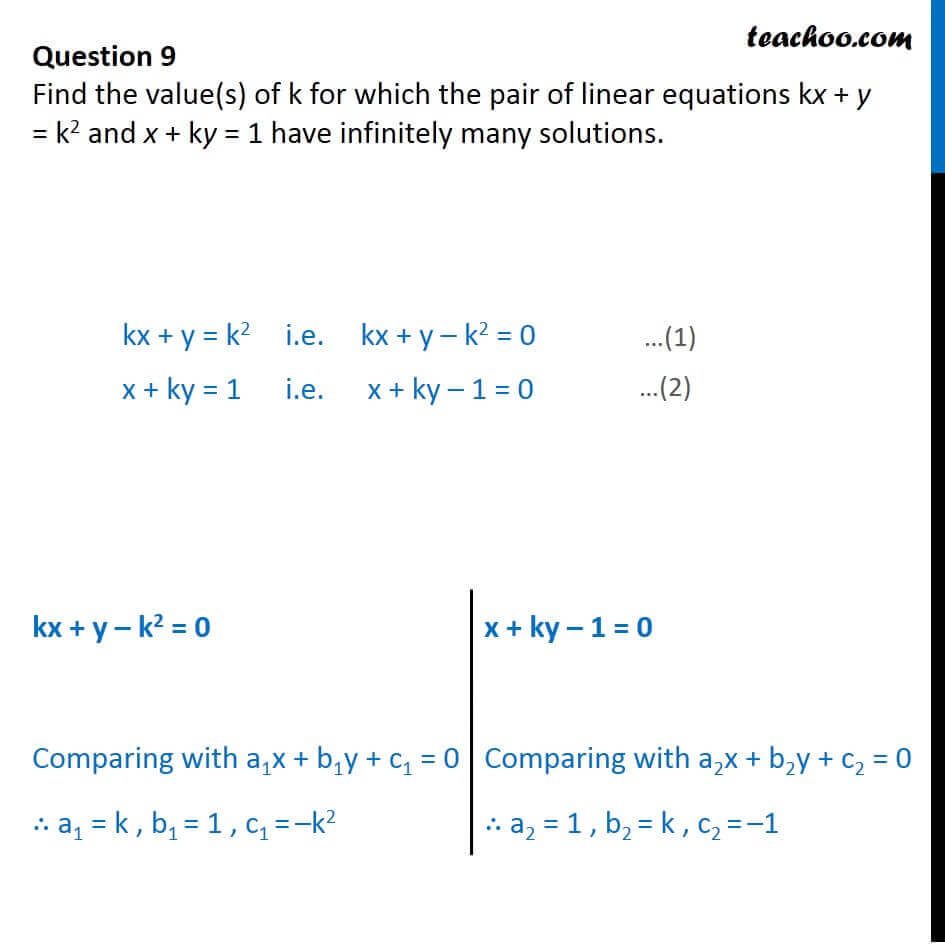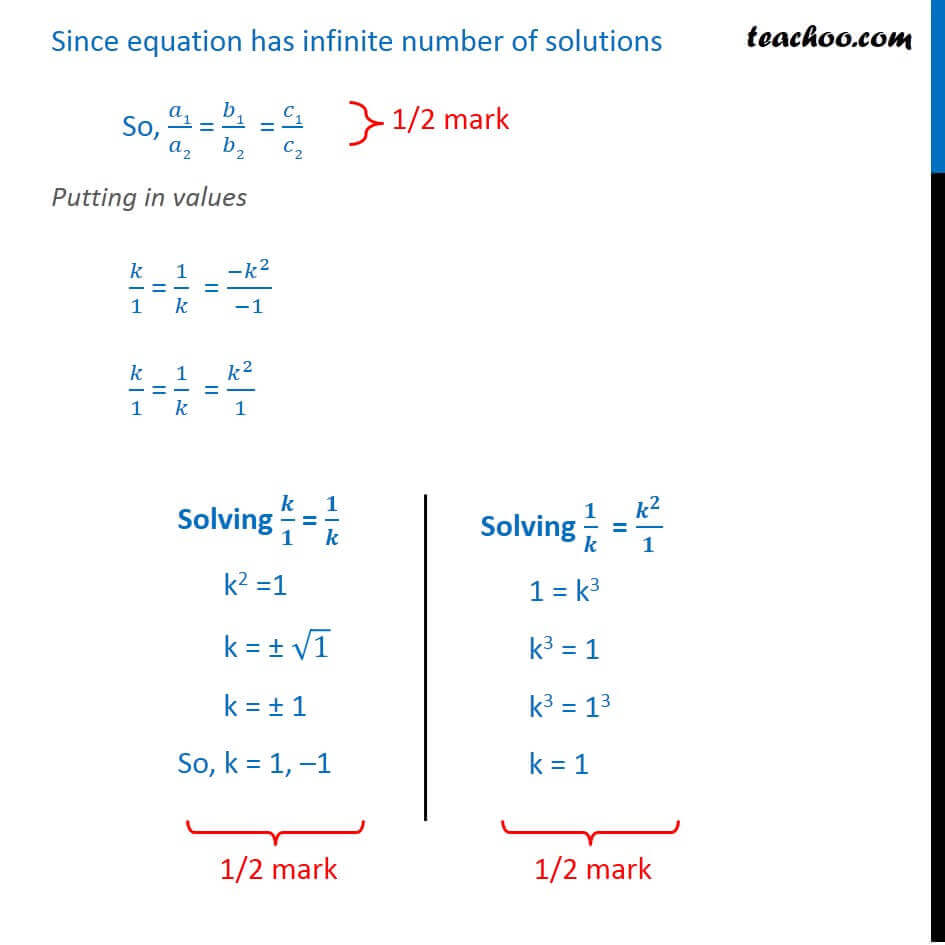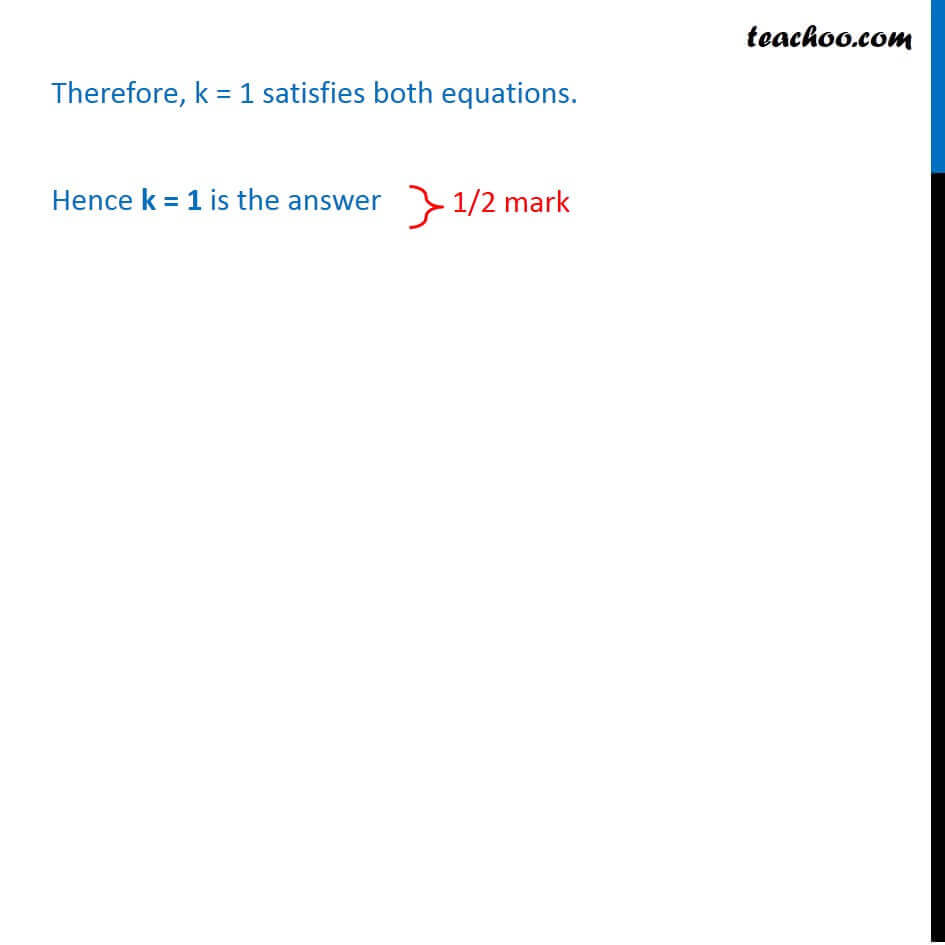CBSE Class 10 Sample Paper for 2018 Boards

Class 10
Solutions of Sample Papers for Class 10 Boards

### Find the value(s) of k for which the pair of linear equations k x + y = k 2 and x + k y = 1 have infinitely many solutions.

This is a question of CBSE Sample Paper - Class 10 - 2017/18.Learn in your speed, with individual attention - Teachoo Maths 1-on-1 Class

### Transcript

Question 9 Find the value(s) of k for which the pair of linear equations kx + y = k2 and x + ky = 1 have infinitely many solutions. kx + y = k2 i.e. kx + y k2 = 0 x + ky = 1 i.e. x + ky 1 = 0 kx + y k2 = 0 Comparing with a1x + b1y + c1 = 0 a1 = k , b1 = 1 , c1 = k2 x + ky 1 = 0 Comparing with a2x + b2y + c2 = 0 a2 = 1 , b2 = k , c2 = 1 Since equation has infinite number of solutions So, 1/ 2 = 1/ 2 = 1/ 2 Putting in values /1 = 1/ = ( ^2)/( 1) /1 = 1/ = ^2/1 Solving / = / k2 =1 k = 1 k = 1 So, k = 1, 1 Solving / = ^ / 1 = k3 k3 = 1 k3 = 13 k = 1 Therefore, k = 1 satisfies both equations. Hence k = 1 is the answer